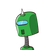# A group of students want to determine if a person’s height is linearly related to the distance they are able to jump.

A group of students want to determine if a person’s height is linearly related to the distance they are able to jump.

To determine the relationship between a person’s height and the distance they are able to jump, the group of students measured the height, in inches, of each person in their class and then measured the distance, in feet, they were able to jump from a marked starting point.

Each student was given three tries at the jump and their longest jump distance was recorded. The data the students collected is shown below.

Height (in.) Jump Distance
(m)
59 5.4
60 5.2
65 6.5
74 6.6
72 6.9
66 6.6
63 6.0
70 6.8
61 5.5
62 5.9
64 6.1
65 6.0
67 6.7
60 5.7
68 6.8
67 6.5

Use a form of technology to compute the correlation coefficient, r, for the linear fit between the person’s height and the distance they were able to jump, where rxy=∑i=1n(xi−x¯¯¯)(yi−y¯¯¯)∑i=1n(xi−x¯¯¯)2∑i=1n(yi−y¯¯¯)2⎷ and n is the number of students and x represents the person’s height and y represents the distance they were able to jump.

1.